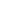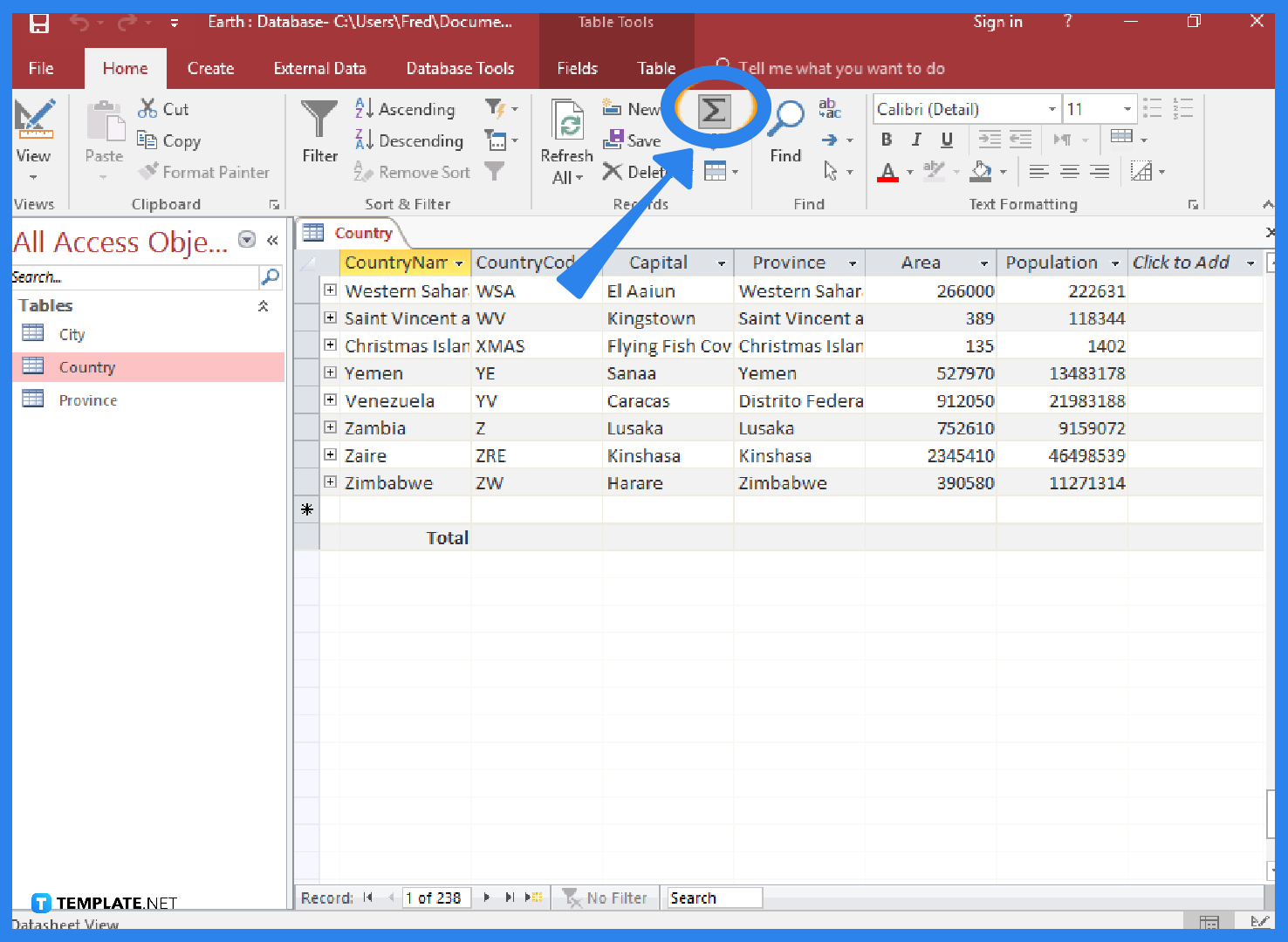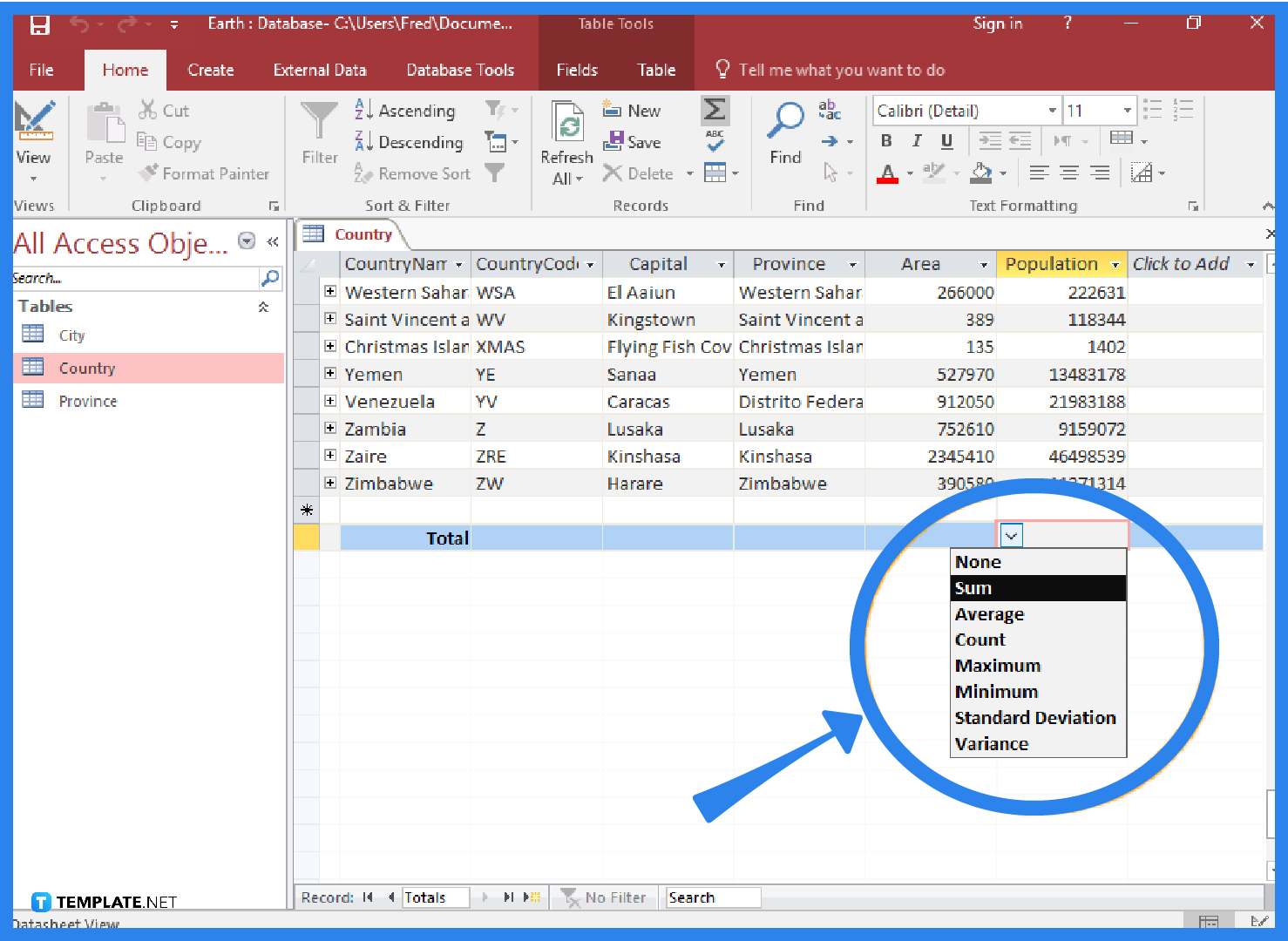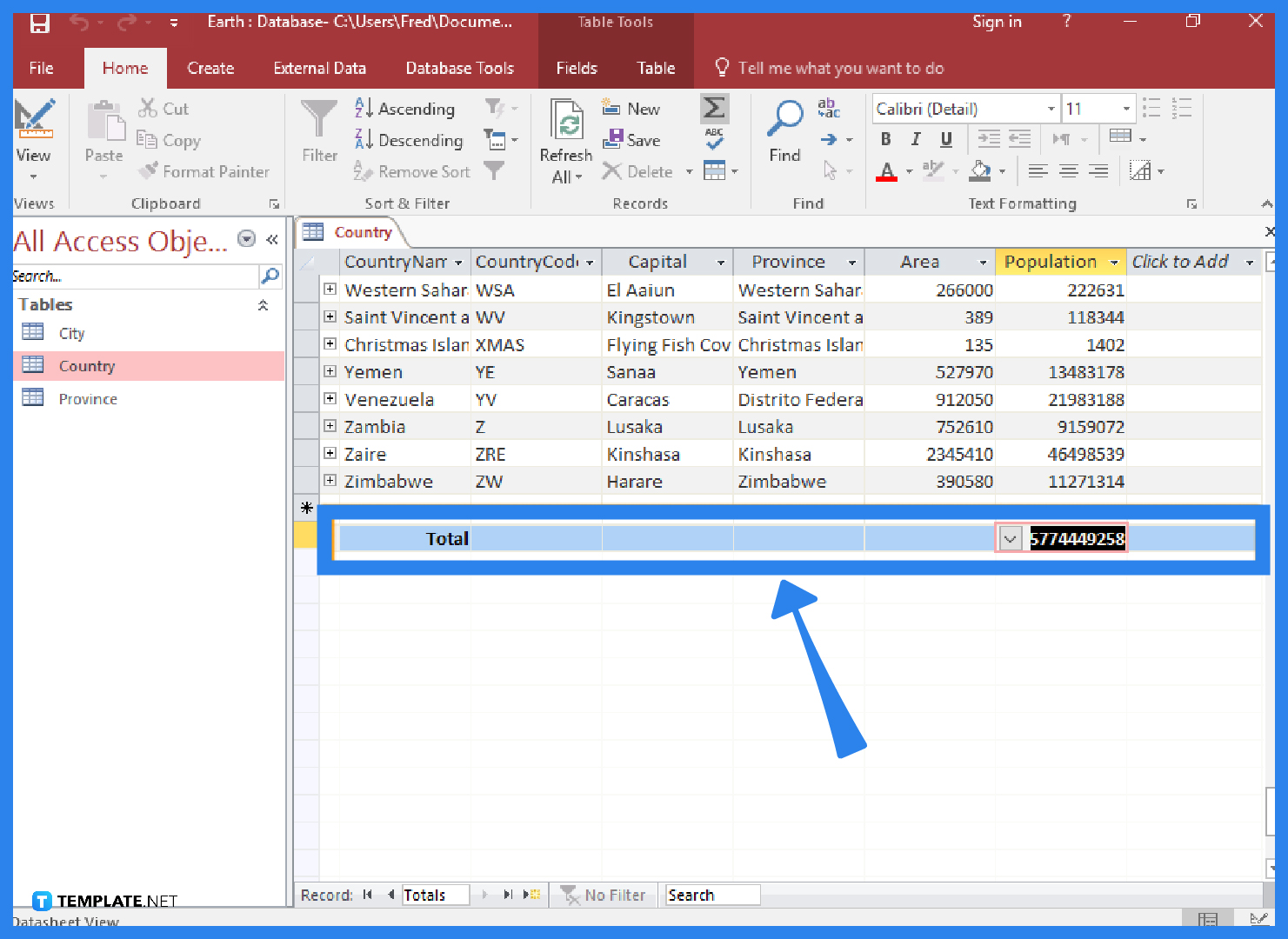# How to Create a Totals Row in Microsoft Access

Microsoft Access‘s totals row enables you to quickly determine the totals for a certain field within a table. Totals rows combine all of the values in the field using an aggregate function, then display the outcome in a separate row.## Download the Free How to Create a Totals Row in Microsoft Access Article in PDFDownload

## How to Create a Totals Row in Microsoft Access

In Microsoft Access, a totals row function can be used to compute the sum of all values, the average of values, the number of records in the table, the minimum value, the highest value, the standard deviation (the degree to which values deviate from a mean or an average), and the variance (statistical variance of all values in the column).

• ### Step 1: Select the Totals Option Under the Home TabClick Totals in the ribbon’s Home tab after opening the table in Datasheet View. The table will be updated with a new row that has the term “Total” in the first column.

• ### Step 2: Choose the Field for the Total and the Desired FunctionClick the field you wish to apply the total to in the new Total row. Then, from the drop-down list, choose the appropriate function, such as sum, average, count, maximum, minimum, standard deviation, and variance. In the example provided in the image, the population field is subjected to the sum function. The population of all nations will be added together, and the result will show up in the new totals row.

• ### Step 3: Get the Totals Row ResultAfter you select the desired option, Microsoft Access will automatically calculate the total and display it on the new totals row.

## FAQs

### Where can I locate the Totals function in Microsoft Access?

You can locate the Totals function of Microsoft Access in the Home tab under the Records Group and it is displayed as a sigma symbol.

### How do I save the changes for the new totals row in Microsoft Access?

Make sure to save the changes you just made to the table if you want the Totals row to remain in place, and to do that, go to the File tab and click on the Save option, or you may also select the Save As option, select the database type, enter the name of your file, choose your preferred file location, and click the Save button.

### What does “total row” mean in a Microsoft Access query?

You can utilize an aggregate function in one or more columns of a query result set using the Total Row feature in Microsoft Access without changing the way your query is constructed; a totals row computes grand totals for one or more columns (fields) of data, whereas a totals query computes subtotals across groups of records.

### How do the calculated fields function and the totals rows function differ in Microsoft Access?

You can make calculations using the data in your tables using calculated fields and totals rows in Microsoft Access; the totals rows function performs a calculation on an entire field of data, whereas the calculated fields function executes calculations using data from a single record.